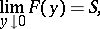# Lambert summation method

(diff) ← Older revision | Latest revision (diff) | Newer revision → (diff)

A method for summing series of numbers. The seriesis summable by Lambert's method to the numberifwhereand if the series on the right-hand side converges. The method was proposed by J.H. Lambert . The summability of a series by the Cesàro summation methodfor some(cf. Cesàro summation methods) to the sumimplies its summability by the Lambert method to the same sum, and if the series is summable by the Lambert method to the sum, then it is also summable by the Abel summation method to the same sum. Lambert's summation method is regular (see Regular summation methods).

How to Cite This Entry:
Lambert summation method. Encyclopedia of Mathematics. URL: http://encyclopediaofmath.org/index.php?title=Lambert_summation_method&oldid=14975
This article was adapted from an original article by I.I. Volkov (originator), which appeared in Encyclopedia of Mathematics - ISBN 1402006098. See original article